# 利用最大似然估计方法估计参数

Matlab2016a

## 实验内容：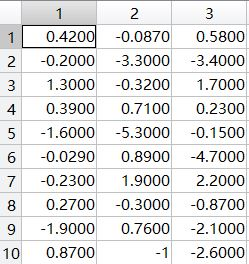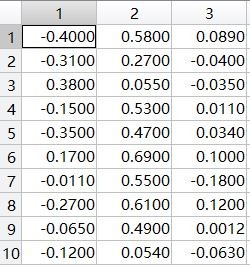（a）编写程序，对表格中的类w1中的3个特征，分别求解最大似然估计。
（b）修改程序，处理二维数据的情形。然后处理对表格中的类w1中的任意两个特征的组合（3种可能）。
（c）修改程序，处理三维数据的情形。然后处理表格中类w1中3个特征的组合。
（d）假设这个三维高斯模型是可分离的，即，写一个程序估计类别w2中均值和协方差矩阵的三个参数。

## 实验步骤：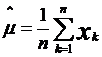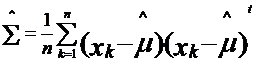load sample_ex3.mat;
x1=w1(:,1);x2=w1(:,2);x3=w1(:,3);
u1=mean(x1);sigma1=mean((x1-u1).^2);
u2=mean(x2);sigma2=mean((x2-u2).^2);
u3=mean(x3);sigma3=mean((x3-u3).^2);
fprintf('the mean and variance of feasure x1: %f ,%f \n',u1,sigma1);
fprintf('the mean and variance of feasure x2: %f ,%f \n',u2,sigma2);
fprintf('the mean and variance of feasure x3: %f ,%f \n',u3,sigma3);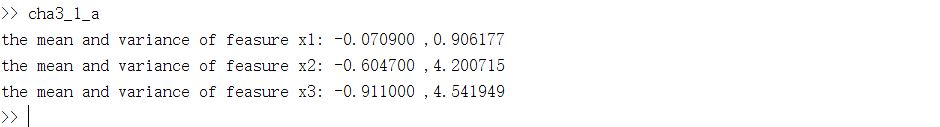load sample_ex3.mat;
x12=[w1(:,1) w1(:,2)];x13=[w1(:,1) w1(:,3)];x23=[w1(:,2) w1(:,3)];
u12=mean(x12',2);u13=mean(x13',2);u23=mean(x23',2);
sum12=0;sum13=0;sum23=0;
for i=1:size(u12,1)
sum12=sum12+(x12(i,:)'-u12)*(x12(i,:)'-u12)';
end
for i=1:size(u13,1)
sum13=sum13+(x13(i,:)'-u13)*(x13(i,:)'-u13)';
end
for i=1:size(u23,1)
sum23=sum23+(x23(i,:)'-u23)*(x23(i,:)'-u23)';
end
sigma12=sum12/size(u12,1);
sigma13=sum13/size(u13,1);
sigma23=sum23/size(u23,1);
disp('the mean and covariance of feasure x1 and x2:');
disp(u12);disp(sigma12);
disp('the mean and covariance of feasure x1 and x3:');
disp(u13);disp(sigma13);
disp('the mean and covariance of feasure x2 and x3:');
disp(u23);disp(sigma23);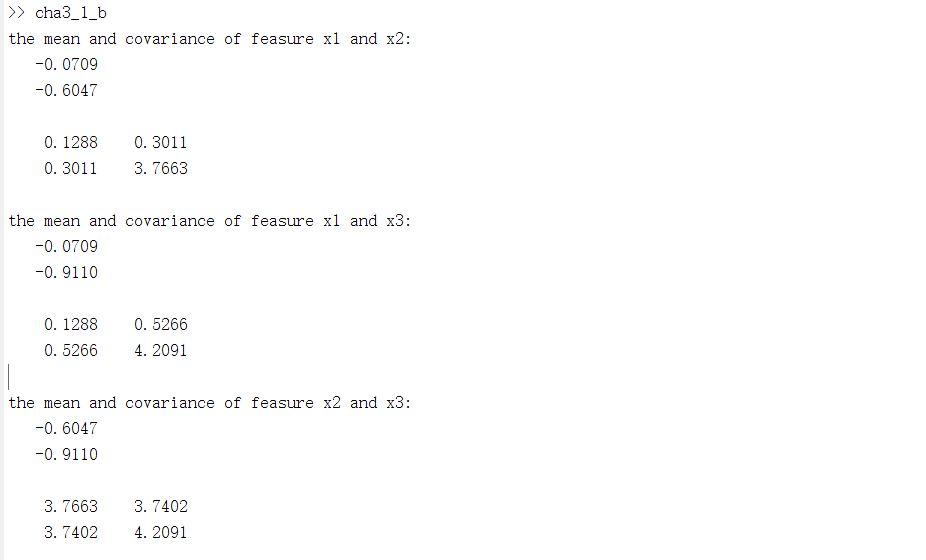clear;
u=mean(w1',2);
sum=0;
for i=1:size(w1,1);
sum=sum+(w1(i,:)'-u)*(w1(i,:)'-u)';
end
sigma=sum/size(w1,1);
disp('the mean and covariance of w1:');
disp(u);disp(sigma);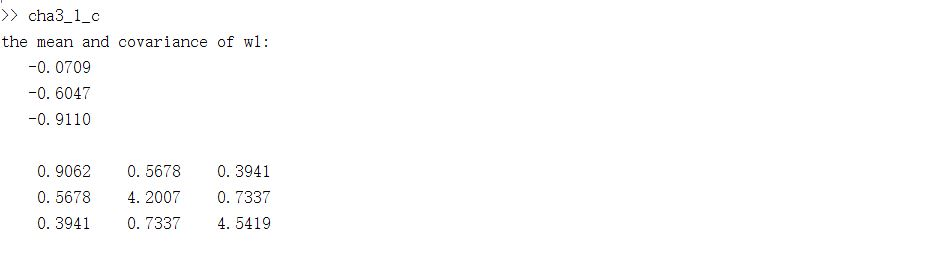load sample_ex3.mat;
x1=w2(:,1);x2=w2(:,2);x3=w2(:,3);
u1=mean(x1);sigma1=mean((x1-u1).^2);
u2=mean(x2);sigma2=mean((x2-u2).^2);
u3=mean(x3);sigma3=mean((x3-u3).^2);
u=[u1;u2;u3];
sigma=[sigma1,0,0;0,sigma2,0;0,0,sigma3];
disp('the mean and covariance of w2:');
disp(u);disp(sigma);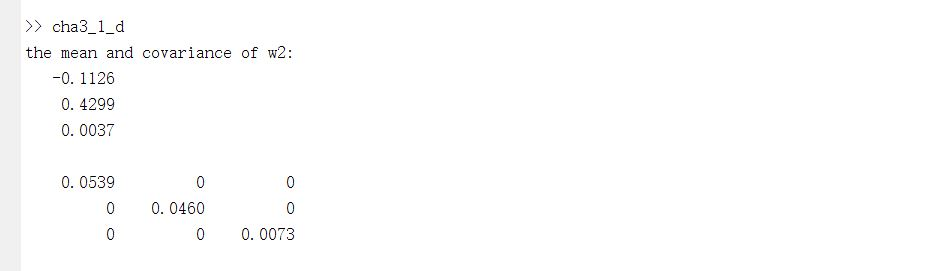## 分析与体会：

12-0503-24
05-213万+
03-235291
01-121万+
09-104万+
08-14153
04-151425
08-10
12-132482
04-281624
04-073375
09-293万+
10-073万+
08-011万+